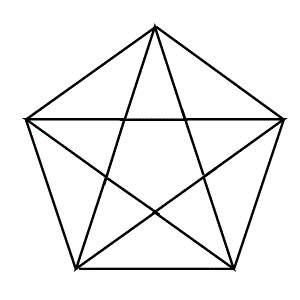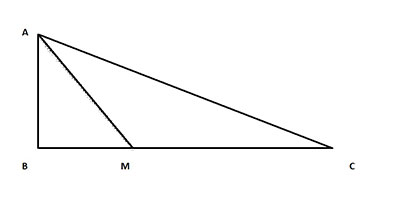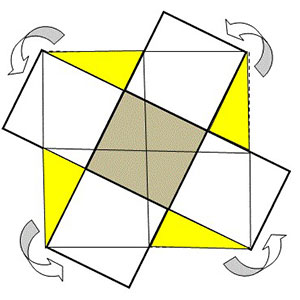# u3a - u3a

Welcome to week 5 of our weekly maths challenge, with problems and puzzles posed by Gordon Burgin, Andrew Holt and the U3A Maths and Stats Subject Adviser - David Martin.

If you would like to share your ideas on how to solve these puzzles please join our learning forum or discuss within your U3A and interest group. The solutions to week two’s problems are below. Check back each week for the solutions - Week 4 solutions are at the bottom of the page - and let us know how you get on by contacting This email address is being protected from spambots. You need JavaScript enabled to view it.

New maths puzzles will go up onto the website every Thursday.

### Week Five

Question 1

Fiona leaves home at 8:30 am on Monday. She averages 60 mph from the time she leaves home until she stops for lunch at 12:00 am. She has travelled 3/5 of the way to her destination. After a 30-minute lunch break, she continues her journey at a slower average rate of 40 mph due to unfavourable weather conditions. What time does she reach her destination?

Question 2

How many triangles of any size are in the following diagrams?Question 3

I have a box without a lid with dimensions 5 x 5 x 5. I place 125 cubes of
unit size in it. How many of these cubes have just one face touching the box?

Question 4
In the right angled triangle below AM bisects the angle at A and meets the side BC at M with BM = 1 cm and MC = 2 cms. What is the length of AM?### Solutions - Week 4

Question 1

In the addition sum below, A, B, C & D represent different digits, and all the digits in the sum are different. What is the sum of A, B, C & D?

Solution

D will be a carry-over from the sum (5 + B = D4), then D = 1.
Since the sum (A + C) cannot be (1 + 2), the only digits available that sum to 13 are 6 and 7 and then B = 14 – 5 – 1 (carry-over) = 8.
The sum of A, B, C & D is (6 + 8 + 7 + 1) = 22.

Question 2

You cash your pay cheque and save half of it. With the rest you pay a debt of £20 and buy something for £5. After spending 1/5 of the remaining money you are left with £12. How much was your pay?

Solution

Let x = pay
Then, pay = ½ (saving) + (debt) + (buy) + 1/5 (remainder) + (left)
x = 1/2x + 20 + 5 + 1/5 (x - 1/2x - 20 - 5) + 12
x = 1/2x + 20 + 5 + 1/5 (1/2x – 25) + 12
x - 1/2x + 1/5 (1/2x) = 25 – 5 +12,
10/10x - 5/10x - 1/10x = 32
4/10x = 32, then x = (32 * 10)/4 = 320 / 4 = £80

Question 3
What is the area of the shaded square formed within a 10cm square?

SolutionArea of the original four 5 cm x 5 cm squares =
10 cm x 10 cm = 100 cm2. By rearranging the 4 segments as shown, four squares at 100 cm2 turn into five squares at 100 cm2. Therefore, the shaded square area (one of the new five squares) is then = 100 / 5 = 20 cm2.

Question 4

I have a wooden right circular cone with height H and radius of base R. What is the greatest volume right circular cylinder I can cut out of this?

Solution
Suppose that the cut is made by removing a cone of height h then the radius of the remaining cylinder is (h/H)R with height H - h. The volume V of the remaining cylinder is therefore given by:
V = π[(h/H)R]2(H-h)
For a maximum or minimum
dV/dh = 0
ie π[(1/H)R]2(2Hh - 3h2) = 0
ie h = 2H/3 and since d2V/dh2 < 0 this is at a maximum
That is, for maximum volume the cylinder must be cut out of the bottom third of the cone giving a volume of:
π[(2/3)R]2H/3 = (4/27)πR2H